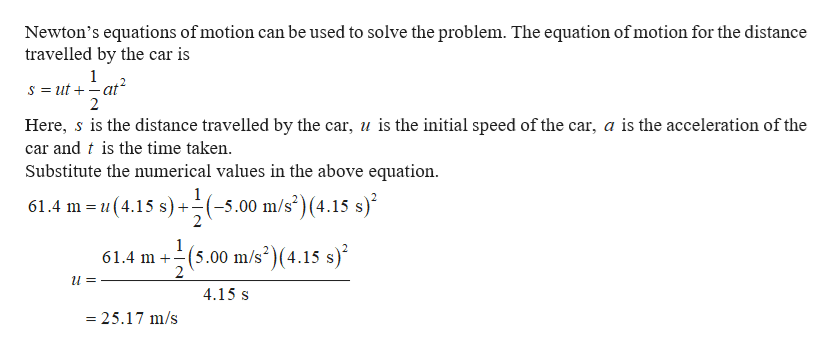The driver of a car slams on the brakes when he sees a tree blocking the road. The car slows uniformly with acceleration of -5.00 m/s2 for 4.15 s, making straight skid marks 61.4 m long, all the way to the tree. With what speed does the car then strike the tree?=?m/s

Question

The driver of a car slams on the brakes when he sees a tree blocking the road. The car slows uniformly with acceleration of -5.00 m/s2 for 4.15 s, making straight skid marks 61.4 m long, all the way to the tree. With what speed does the car then strike the tree?

=?m/s

Step 1help_outlineImage TranscriptioncloseNewton's equations of motion can be used to solve the problem. The equation of motion for the distance travelled by the car is 1 S ut at 2 = Here, s is the distance travelled by the car, u is the initial speed of the car, a is the acceleration of the car and t is the time taken Substitute the numerical values in the above equation 1 61.4 m u(4.15 s) -5.00 m/s)(4.15 s) 1 61.4 m 2 (5.00 m/s2)(4.15 s) 4.15 s 25.17 m/s fullscreen

Want to see the full answer?

See Solution

Want to see this answer and more?

Our solutions are written by experts, many with advanced degrees, and available 24/7

See Solution
Tagged in

Newtons Laws of Motion